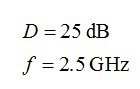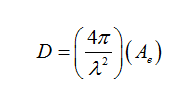# If the directivity of an antenna is 25 dB at 2.5 GHz, then what will be the effective aperture of the antenna?

Question
1 views

If the directivity of an antenna is 25 dB at 2.5 GHz, then what will be the effective aperture of the antenna?

check_circle

Step 1

The given values of directivity and frequency are:Formula:

The following formula is used to determine the effective aperture(Ae):Step 3

The wavelength (λ) is determined as follows:

...

### Want to see the full answer?

See Solution

#### Want to see this answer and more?

Solutions are written by subject experts who are available 24/7. Questions are typically answered within 1 hour.*

See Solution
*Response times may vary by subject and question.
Tagged in

### Electrical Engineering